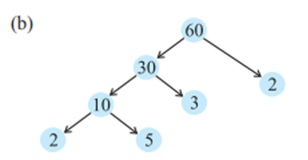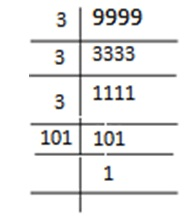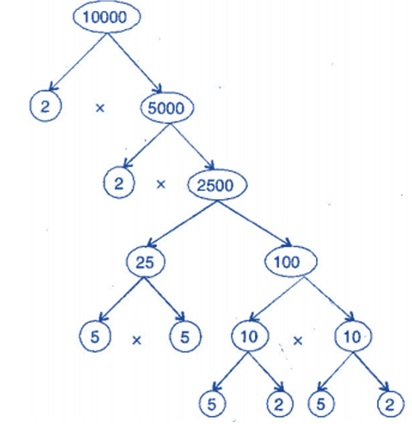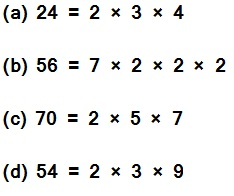Study Materials: ncert solutions

Our ncert solutions for Exercise 3.5 Class 6 maths 3. Playing with Numbers - ncert solutions - Toppers Study is the best material for English Medium students cbse board and other state boards students.

Solutions ⇒ Class 6th ⇒ Mathematics ⇒ 3. Playing with Numbers

# Exercise 3.5 Class 6 maths 3. Playing with Numbers - ncert solutions - Toppers Study

Topper Study classes prepares ncert solutions on practical base problems and comes out with the best result that helps the students and teachers as well as tutors and so many ecademic coaching classes that they need in practical life. Our ncert solutions for Exercise 3.5 Class 6 maths 3. Playing with Numbers - ncert solutions - Toppers Study is the best material for English Medium students cbse board and other state boards students.

## Exercise 3.5 Class 6 maths 3. Playing with Numbers - ncert solutions - Toppers Study

CBSE board students who preparing for class 6 ncert solutions maths and Mathematics solved exercise chapter 3. Playing with Numbers available and this helps in upcoming exams 2023-2024.

### You can Find Mathematics solution Class 6 Chapter 3. Playing with Numbers

• All Chapter review quick revision notes for chapter 3. Playing with Numbers Class 6
• NCERT Solutions And Textual questions Answers Class 6 Mathematics
• Extra NCERT Book questions Answers Class 6 Mathematics
• Importatnt key points with additional Assignment and questions bank solved.

NCERT Solutions do not only help you to cover your syllabus but also will give to textual support in exams 2023-2024 to complete exercise 3.5 maths class 6 chapter 3 in english medium. So revise and practice these all cbse study materials like class 6 maths chapter 3.5 in english ncert book. Also ensure to repractice all syllabus within time or before board exams for ncert class 6 maths ex 3.5 in english.

See all solutions for class 6 maths chapter 3 exercise 3 in english medium solved questions with answers.

### Exercise 3.5 class 6 Mathematics Chapter 3. Playing with Numbers

• Exercise 3.5 Class 6 Maths 3. Playing With Numbers - Ncert Solutions - Toppers Study
• Class 6 Ncert Solutions
• Solution Chapter 3. Playing With Numbers Class 6
• Solutions Class 6
• Chapter 3. Playing With Numbers Exercise 3.5 Class 6

## Exercise 3.5 Class 6 maths 3. Playing with Numbers - ncert solutions - Toppers Study

Exercise 3.5

1. Which of the following statements are true?

(a) If a number is divisible by 3, it must be divisible by 9.

(b) If a number is divisible by 9, it must be divisible by 3.

(c) A number is divisible by 18, if it is divisible by both 3 and 6.

(d) If a number is divisible by 9 and 10 both, then it must be divisible by 90.

(e) If two numbers are co-primes, at least one of them must be prime.

(f) All numbers which are divisible by 4 must also be divisible by 8.

(g) All numbers which are divisible by 8 must also be divisible by 4.

(h) If a number exactly divides two numbers separately, it must exactly divide their sum.

(i) If a number exactly divides the sum of two numbers, it must exactly divide the two numbers separately.

2. Here are two different factor trees for 60. Write the missing numbers.

Solution:

There are two different way as follow:3. Which factors are not included in the prime factorization of a composite number?

Solution: 1 and the composite number itself not included in the prime factorization of a composite number.

4. Write the greatest 4-digit number and express it in terms of its prime factors.

Solution: The greatest 4-digit number -5. Write the smallest 5-digit number and express it in the form of its prime factors.

Solution:

The smallest five diigit number is 10000.

It's tree factor is :Hence the prime factorisation =

2 × 2 × 2 × 2 × 5 × 5 × 5 × 5

6. Find all the prime factors of 1729 and arrange them in ascending order. Now state the relation, if any; between two consecutive prime factors.

Solution:

Prime factors of 1729 are 7 × 13 × 19.7. The product of three consecutive numbers is always divisible by 6. Verify this statement with the help of some examples.

Solution:  Among the three consecutive numbers, there must be one even number and one multiple of 3. Thus, the product must be multiple of 6.

Example:

(i) 2 × 3 × 4 = 24

(ii) 4 × 5 × 6 = 120

8. The sum of two consecutive odd numbers is divisible by 4. Verify this statement with the help of some examples.

Solution: 3 + 5 = 8 and 8 is divisible by 4.

5 + 7 = 12 and 12 is divisible by 4.

7 + 9 = 16 and 16 is divisible by 4.

9 + 11 = 20 and 20 is divisible by 4.

9. In which of the following expressions, prime factorisation has been done?Solution:  In expressions (b) and (c), prime factorization has been done.

10. Determine if 25110 is divisible by 45.

[Hint: 5 and 9 are co-prime numbers. Test the divisibility of the number by 5 and 9].

Solution: The prime factorization of 45 = 5 × 9 25110 is divisible by 5 as ‘0’ is at its unit place.

25110 is divisible by 9 as sum of digits is divisible by 9.

Therefore, the number must be divisible by 5 × 9 = 45

11. 18 is divisible by both 2 and 3. It is also divisible by 2 × 3 = 6. Similarly, a number is divisible by both 4 and 6. Can we say that the number must also be divisible by 4 × 6 = 24? If not, give an example to justify your answer.

Solution: No. Number 12 is divisible by both 6 and 4 but 12 is not divisible by 24.

12. I am the smallest number, having four different prime factors. Can you find me?

Solution: The smallest four prime numbers are 2, 3, 5 and 7.

Hence, the required number is 2 × 3 × 5 × 7 = 210

Important Study materials for classes 06, 07, 08,09,10, 11 and 12. Like CBSE Notes, Notes for Science, Notes for maths, Notes for Social Science, Notes for Accountancy, Notes for Economics, Notes for political Science, Noes for History, Notes For Bussiness Study, Physical Educations, Sample Papers, Test Papers, Mock Test Papers, Support Materials and Books.Mathematics Class - 11th

NCERT Maths book for CBSE Students.

books

## Study Materials List:

##### Solutions ⇒ Class 6th ⇒ Mathematics
1. Knowing Our Numbers
2. Whole Numbers
3. Playing with Numbers
4. Basic Geometrical Ideas
5. Understanding Elementary Shapes
6. Integers
7. Fractions
8. Decimals

New Books